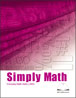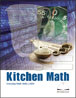# Everyday Math Skill

## Simply Math (2009)This math skills booklet is designed to help adult learners with math operations. It is to be used with the three workbooks in the NWT Literacy Council's Everyday Math Skills series - Money Math, Kitchen Math and Home Math. Written in plain language, it covers basic math skills, fractions, decimals, percentages, metric units, and measurements.

2010-01-13

## Money Math (2009)Money Math is one of three workbooks in the NWT Literacy Council's Everyday Math Skills series. It can be used in conjunction with the math booklet, Simply Math. Money Math is designed to help adult learners with math and is written in plain language. It is divided into three sections, each covering a variety of math concepts, followed by worksheets and a review page.

2010-01-13

## Kitchen Math (2009)Kitchen Math is one of three workbooks in the NWT Literacy Council's Everyday Math Skills series. It can be used in conjunction with the math booklet, Simply Math. Kitchen Math is designed to help adult learners with math and is written in plain language. It is divided into three sections, each covering a variety of math concepts, followed by worksheets and a review page.